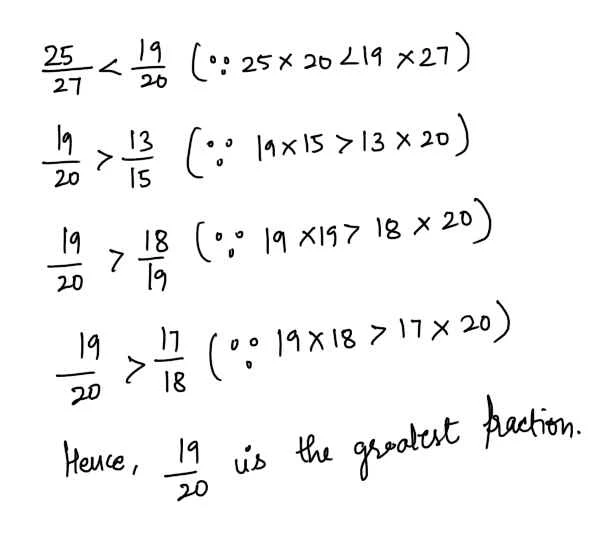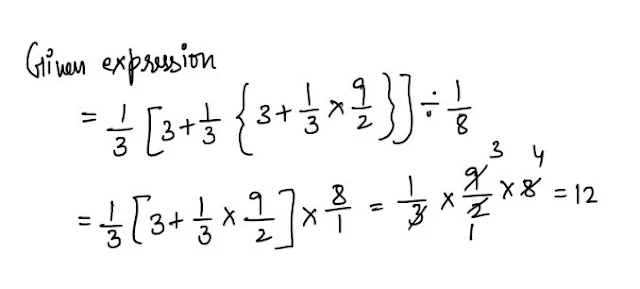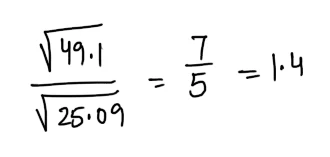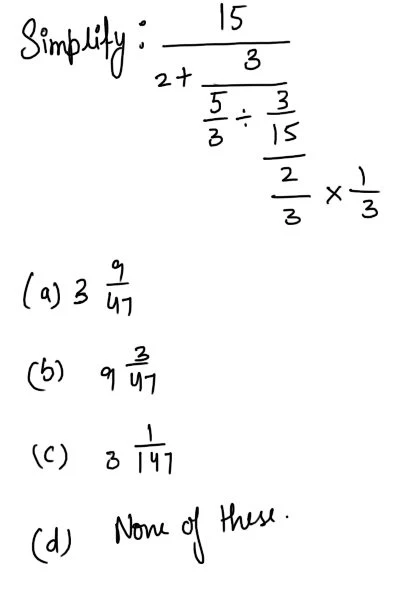New Student User - Use Code HELLO

# Quant Quiz For IBPS PO Pre Part - 13#### Ques 1.

1.333 + 1.25/1.333 -1.25 is nearly equal to
(a) 13
(b) 31
(c) 21
(d) 33
Ans 1.#### Ques 2.

Out of 25/27, 19/20, 13/15, 18/19, 17/18 which is the greatest in magnitude ?
(a)25/27
(b) 19/20
(c) 13/15
(d) 17/18
Ans 2.#### Ques 3.

[ 4-5 + { 1-(4 -3 +1)}] of 100% equals
(a) 50%
(b) -1%
(c) zero
(d) 100%
Ans 3.#### Ques 4.

Value of 1//8 is nearly
(a) .365
(b) .353
(c) .25
(d) None of these
Ans 4.#### Ques 5.

1/3 [ 3 + 1/3 (3 +1 1/2)}] -1/8 equals
(a) 12
(b) 3/16
(c) 6
(d) None of these
Ans 5#### Ques 6.

80.6251 x 0.6317 is nearly equal to
(a) 51
(b) 55
(c) 59
(d) None of these
Ans  6.#### Ques 7

/49.1 ÷ 0.6317 is nearly equals
(a) 1.6
(b) 1.55
(c) 1.4
(d) 1.35
Ans 7.#### Ques 8.Ans 8.#### Ques 9.

The least number of five digits exactly divisible by 654 is
(a) 10000
(b) 10654
(c) 10464
(d) None of these
Ans 9. The least number of 5 digits is 10000. On dividing 10000 by 654 the remainder is 190.
Hence, the required number is 10000 + (654 - 190) i.e. 10464.

#### Ques 10.

The L.C.M and H.C.F. of two numbers are 4284 and 34 respectively .If one number id 204,find the second number.
(a)744
(b) 714
(c) 340
(d) 428
Ans 10.